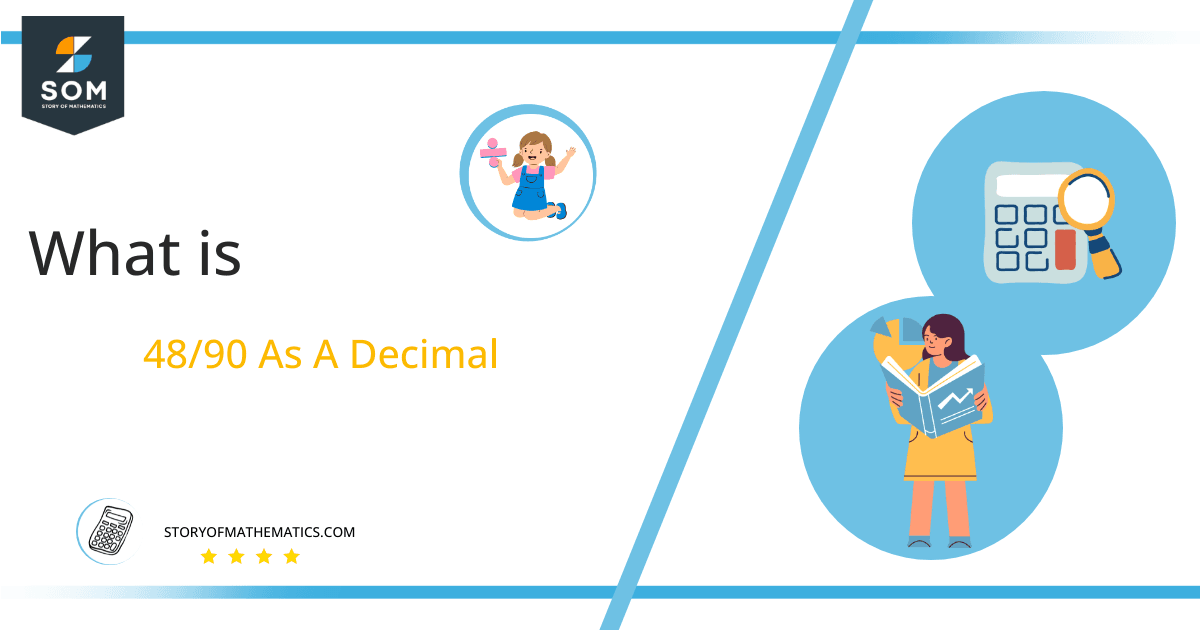# What Is 48/90 as a Decimal + Solution With Free Steps

The fraction 48/90 as a decimal is equal to 0.53333333.

A Division operator is used for converting Fractional quantities to Decimal values. The fraction’s upper portion is referred to as the Numerator, and its lower portion is known as the Denominator.Here, we are more interested in the division types that result in a Decimal value, as this can be expressed as a Fraction. We see fractions as a way of showing two numbers having the operation of Division between them that result in a value that lies between two Integers.

Now, we introduce the method used to solve said fraction to decimal conversion, called Long Division, which we will discuss in detail moving forward. So, let’s go through the Solution of fraction 48/90.

## Solution

First, we convert the fraction components, i.e., the numerator and the denominator, and transform them into the division constituents, i.e., the Dividend and the Divisor, respectively.

This can be done as follows:

Dividend = 48

Divisor = 90

Now, we introduce the most important quantity in our division process: the Quotient. The value represents the Solution to our division and can be expressed as having the following relationship with the Division constituents:

Quotient = Dividend $\div$ Divisor = 48 $\div$ 90

This is when we go through the Long Division solution to our problem.Figure 1

## 48/90 Long Division Method

We start solving a problem using the Long Division Method by first taking apart the division’s components and comparing them. As we have 48 and 90, we can see how 48 is Smaller than 90, and to solve this division, we require that 48 be Bigger than 90.

This is done by multiplying the dividend by 10 and checking whether it is bigger than the divisor or not. If so, we calculate the Multiple of the divisor closest to the dividend and subtract it from the Dividend. This produces the Remainder, which we then use as the dividend later.

Now, we begin solving for our dividend 48, which after getting multiplied by 10 becomes 480.

We take this 480 and divide it by 90; this can be done as follows:

480 $\div$ 90 $\approx$ 5

Where:

90 x 5 = 450

This will lead to the generation of a Remainder equal to 480 – 450 = 30. Now this means we have to repeat the process by Converting the 30 into 300 and solving for that:

300 $\div$ 90 $\approx$ 3

Where:

90 x 3 = 270

This, therefore, produces another Remainder which is equal to 300 – 270 = 30.

Finally, we have a Quotient generated after combining the pieces of it as 0.53=z, with a Remainder equal to 30.Images/mathematical drawings are created with GeoGebra.